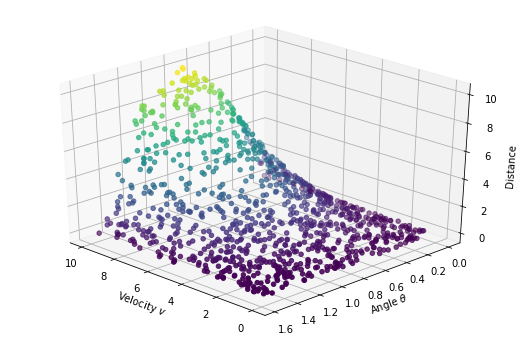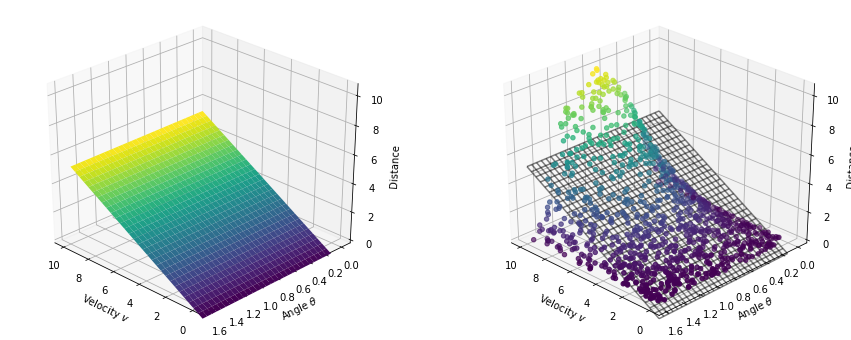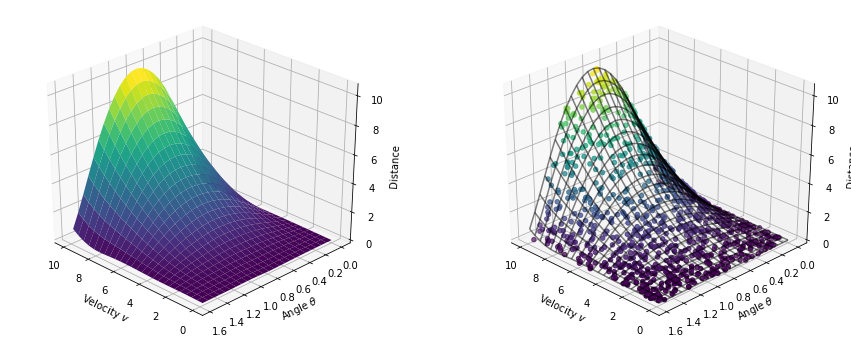Having spent my academic career so far purely within mathematics I have been well experienced in creating and analysing mathematical and physical models of the world around us through the use of differential equations and probability theory. However through my interactions with industry (from small start-ups to international giants) I have found that companies are more often than not looking for a 'machine learning' approach, whether it is suitable or not. This is echoed in industry as a whole as businesses adopt machine learning into their culture and organisation.

Interestingly the machine learning buzz is also spilling over into mathematics, at both undergraduate and postgraduate levels. The Masters option "Theories of Deep Learning" here at Oxford has been massively oversubscribed in it's maiden year, highlighting the demand for such a course, especially when compared to other comparable courses on optimisation. This is understandable of course - with employers seeking out graduates with generic data science skills then such courses are a stepping stone to becoming an appealing prospect, especially with the current skill gap in this area. Perhaps more surprisingly I'm seeing doctoral students looking to incorporate machine learning into their research, leading to novel hybrid models combining the 'traditional' (ODEs, PDEs) with the 'modern' (Deep Learning) - more on this later!

So just where does machine learning fit in to the scientific world and are we eventually going to have to resort to such methods to make progress in modelling if mathematical models cannot keep up? I'd like to argue that machine learning and mathematical models can coexist nicely in both research and industrial applications, and that the most interesting models appear when we utilise the power of both paradigms. To illustrate this point we take a trip to a civilisation who are in the contradictory position of having invented machine learning (implemented in PyTorch, strangely...) but their mathematics is not up to scratch. In particular they are not familiar with modern calculus. This example should hopefully replicate and illuminate the situation we find ourselves in now - where mathematics is difficult but data collection is easy.

What this post is:

• An examination of where machine learning fits into the scientific toolkit
• A showcase of tools and methods for solving a 'simple' problem
• An attempt to convince you to think about how best to model a problem

What this post is not:

• A step by step guide to machine learning
• Best practise for implementing machine learning models
• A criticism of machine learning
• A numerical evaluation/comparison of methods

## A Quasi-Historical Example¶Suppose we are in a pre-calculus, post-deep-learning civilisation. Like most civilisations, they are interested in ways to destroy one another. Two scientists from one tribe are tasked with modelling the range of a new cannon they have just invented. The scientists can control:

1. the amount of gunpower they load the cannon with (i.e, the velocity of the projectile)
2. the angle at which they point the cannon

The scientists can measure:

1. the straight-line distance the cannonball travels from the cannon

Note: Here we assume that the ground is completely level.

Mathematically speaking, they want to find a model/function $F$ such that for any velocity $v$ and angle $\theta$, they can produce a prediction $$s = F(v,\theta)$$ which is close to the true distance travelled.

With no prior knowledge about how projectiles move through the air the scientists resort to a data-driven approach.

### Data Collection¶

The scientists have been given a day to fire the cannon as many times as they can with a range of different angles and firepower. Each time they fire they cannon they measure the distance from the starting point to where the cannonball ends. Their measurement is not without error however - each measurement is subject to an amount of additive noise (so that the measurement error is not proportional to the distance travelled).

Over the course of the day they manage to fire the cannon 1000 times, resulting in a dataset of 1000 triples $(v_i, \theta_i, s_i)$, where $\theta_i$ is recorded in radians.

In :
# Generating a dataset.
vs = 10*np.random.random(size=1000)[:,np.newaxis]
thetas = np.pi/2*np.random.random(size=1000)[:,np.newaxis]
g = 9.81
distances = (vs**2)*np.sin(2*thetas)/g + 0.01*np.random.randn(thetas.shape)[:,np.newaxis]
distances[distances<0] = 0

# PyTorch Tensors
x_train = torch.from_numpy(np.hstack([vs,thetas]).astype(np.float32))
y_train = torch.from_numpy(distances.astype(np.float32))


They arrive at the following plot:### 1. A Model-less Approach¶

The simplest approach to the problem is to not have a model at all - the data is the model! In this approach they pick the closest historical data they have to the data they want to predict and use the output of the historical data as their prediction, i.e. $$F(v,\theta) = s_{i^*} \quad \text{ where } \quad i^* = \textrm{argmin}_{i} \{\textrm{Dist}((v,\theta), (v_i,\theta_i)) \}$$ where $\textrm{Dist}(\cdot, \cdot)$ is a distance function such as the L2-norm.

In :
# A model-less approach: find the closest data point we have an return the
# resulting output.

def closest_node(node, nodes):
nodes = np.asarray(nodes)
dist_2 = np.sum((nodes - node)**2, axis=1)
return np.argmin(dist_2)

# Give a prediction for the point (4.0,1.2)
ix = closest_node([4,1.2], x_train)
x_train[ix,:], y_train[ix]

Out:
(tensor([3.9268, 1.2042]), tensor([1.0437]))

This purely-data driven approach has an obvious draw-back. If their data does not cover the range of possible inputs, or the data is sparse, then they can run into issues. Here, without a model they cannot accurately predict what would happen if they considered velocities greater than $v=10$ for example.

In :
# Give a prediction for the point (20.0,0.85)
ix = closest_node([20,0.85], x_train)
x_train[ix,:], y_train[ix]

Out:
(tensor([9.9885, 1.1096]), tensor([8.1159]))

Despite this drawn-back, the model-less approach does see use in industry, in particular to address the cold start problem. For example, recommendation systems (e.g. video recommendation on Netflix, product recommendations at Amazon) can be incredibly powerful tools to learn your preferences however these rely on having at least some information about your preferences. For new customers this information is not present. One approach therefore is to take whatever information is present (e.g. age, location, gender) and match initial predictions to those of an existing customer.

### 2. A Linear Model Approach¶

Looking at the data it is clear that their function is non-linear, and a linear model is unlikely to be accurate. It can however sometimes be difficult to visualise the shape of data, or such analysis is omitted altogether! Linear models are not without merit and are fundamental to many applications, and so they decide to try using one.

The linear model is described by $$F(v, \theta) = w_1v + w_2\theta +b$$ where $w_i\in \mathbb{R}$ are weights and $b\in \mathbb{R}$ is a bias term, all to be determined. For continuity they implement the linear model in PyTorch and find the model parameters using stochastic gradient descent (there are of course cleverer and simpler methods).

In :
# A simple linear regression model implemented in PyTorch.
class LinearRegressor(nn.Module):

def __init__(self, input_dim, output_dim):
""" A two layer network mapping input directly to output. """

super(LinearRegressor, self).__init__()
self.linear = nn.Linear(input_dim, output_dim)

def forward(self, x):
return self.linear(x)

def get_parameters(self):
""" Returns the model weights and bias. """
w, b = self.parameters()
return w.data, b.data

In :
# Initialise the model, assign a loss function, and optimiser.
model = LinearRegressor(2,1)
criterion = nn.MSELoss()
optimizer = optim.SGD(model.parameters(), lr = 0.01)

In :
# Run the model
num_epochs = 500
for epoch in range(num_epochs):

# Forward pass: Compute predicted y by passing x to the model
pred_y = model.forward(x_train)

# Compute loss
loss = criterion(pred_y, y_train)

# Zero gradients, perform a backward pass,
# and update model weights.
loss.backward()
optimizer.step()

if (epoch+1) % 100 == 0:
print('Epoch[{}/{}], Loss: {:.6f}'.format(epoch+1, num_epochs, loss.data))

Epoch[100/500], loss: 2.426076
Epoch[200/500], loss: 2.393808
Epoch[300/500], loss: 2.389446
Epoch[400/500], loss: 2.388700
Epoch[500/500], loss: 2.388478As expected, the linear model fails to capture both the non-monotonicity in the $\theta$-direction, and the quadratic grow in the $v$-direction.

### 3. The Black-box Approach - Deep neural networks¶

Having invested heavily in machine learning research and computational frameworks, the scientists are keen to throw the metaphorical kitchen sink at the problem, in the form of a deep neural network. Specifically they utilise a multilayer perceptron (MLP) which consists of number of linear layers with a non-linear activation interspersed between. The model can be described by $$\textrm{Linear}(2,256) \to \textrm{Sigmoid} \to \textrm{Linear}(256,256) \to \textrm{Sigmoid} \to \textrm{Linear}(256,1)$$ where the arguments represent the number of variables each model takes, i.e. two inputs and one output. There are many natural questions to ask here - how deep or wide does the network need to be and which activation functions should be used? Furthermore how quickly does this network train and does it approach a global optimal solution? These questions form a significant part of current research in machine learning, and fortunately some of them have answers.

In :
# "Black Box" Neural Network, or MultiLayer Perceptron (MLP)
class Blackbox(nn.Module):

def __init__(self, n_feature, n_hidden, n_output):
super().__init__()
self.hidden1 = torch.nn.Linear(n_feature, n_hidden)
self.hidden2 = torch.nn.Linear(n_hidden, n_hidden)
self.predict = torch.nn.Linear(n_hidden, n_output)

def forward(self, x):
x = torch.sigmoid(self.hidden1(x))
x = torch.sigmoid(self.hidden2(x))
x = self.predict(x)
return x

In :
x_train = torch.from_numpy(np.hstack([vs,thetas]).astype(np.float32))
y_train = torch.from_numpy(distances.astype(np.float32))

# Initialise the model, loss function, and optimiser (Adam).
model = Blackbox(n_feature=2, n_hidden=256, n_output=1)
criterion = nn.MSELoss()

# Use a GPU if available to speed up training
device = torch.device("cuda" if torch.cuda.is_available() else "cpu")
print("Using {}".format(device))
model = model.to(device)

Using cuda

In :
# Train the model
num_epochs = 1000
losses = []
for epoch in range(num_epochs):

# Forward pass
pred_y = model.forward(x_train.to(device))

# Compute loss
loss = criterion(pred_y, y_train.to(device))

# Zero gradients, perform a backward pass,
# and update the weights.
loss.backward()
optimizer.step()

if (epoch+1) % 200 == 0:
losses.append(loss.data)
print('Epoch[{}/{}], Loss: {:.6f}, Max Gradient: {:.6f}'
.format(epoch+1, num_epochs, loss.data, max([(-x.data).max() for x in model.parameters()])))

Epoch[200/1000], Loss: 0.111930, Max Gradient: 2.746020793914795
Epoch[400/1000], Loss: 0.032923, Max Gradient: 2.7668488025665283
Epoch[600/1000], Loss: 0.008271, Max Gradient: 2.79529070854187
Epoch[800/1000], Loss: 0.002359, Max Gradient: 2.824122190475464
Epoch[1000/1000], Loss: 0.001108, Max Gradient: 2.8465383052825928


The model is again trained using an adaptive optimiser called Adam, giving the function below.For the uninitiated it might be surprising how well the neural network approximates the given data. This has predominantly been the story of deep learning so far - it works, and can work extremely well, but we are not always sure why.

### 4. The Quasi-linear Approach - Learning a mathematical expression¶

In the black-box model the scientists have a model that can accurately predict how far the cannon is going to fire, however as the name suggests, they have no intuition to the form of the function. The scientists are keen to get this intuition back while still using a machine learning approach and resort back to using a linear model.

Having studied trigonometry in their school days, the scientists are convinced that the solution to the problem will likely involve some trigonometric functions of the angle composed with a function of the velocity. They propose to write the model as $$F(v,\theta) = w_1 v + w_2 v^2 + w_3 v\sin(\theta) + w_4 v\sin(2\theta) + w_5 v^2 \sin(\theta) + w_6 v^2 \sin(2\theta),$$ a linear combination of non-linear basis functions.

In :
# Linear regression using a set of basis functions.

class BasisRegressor(nn.Module):
"""Approximates a set of points by a set of basis functions."""

def __init__(self, basis):
super(BasisRegressor, self).__init__()
self.basis = basis
self.weights = nn.Parameter(torch.Tensor(len(basis)))
self.weights.data = torch.from_numpy(np.random.random(len(basis))[:np.newaxis].astype(np.float32))

def forward(self, x):
prediction = torch.stack([w*f(x[:,0],x[:,1]) for w,f in zip(self.weights,self.basis)])
prediction = torch.sum(prediction, dim=0)
return prediction.unsqueeze(1)

def get_parameters(self):
weights, = self.parameters()
return weights.data

In :
# Initialise the model using basis function list
# Set the loss function and optimiser.
basis_functions = [lambda x,y: x,
lambda x,y: x**2,
lambda x,y: x*np.sin(y),
lambda x,y: x*np.sin(2*y),
lambda x,y: (x**2)*np.sin(y),
lambda x,y: (x**2)*np.sin(2*y)]

model = BasisRegressor(basis_functions)
criterion = nn.MSELoss()

In :
num_epochs = 5000
for epoch in range(num_epochs):

# Forward pass: Compute predicted y by passing x to the model
pred_y = model.forward(x_train)

# Compute loss
loss = criterion(pred_y, y_train)

# Zero gradients, perform a backward pass,
# and update the weights.
loss.backward()
optimizer.step()

if (epoch+1) % 1000 == 0:
print('Epoch[{}/{}], Loss: {:.6f}, Max Gradient: {:.6f}'

Epoch[1000/5000], Loss: 0.010195, Max Gradient: 0.054714

$$x^* = 0.00v + -0.00v^2 + -0.00v\sin(\theta) + 0.00v\sin(2\theta) + 0.00v^2\sin(\theta) + 0.10v^2\sin(2\theta)$$